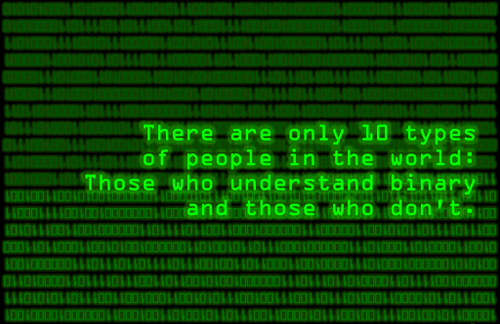“不行你再数数”

“怎么数？我手指都用完了怎么数？要不你借我几根？”

“借你就借你，借几根手指能怀孕还是怎么着。”爸爸三号说，“不过我的手指跟你的可不一样，我这一根手指顶你的十根。我掰一根手指，就表示你十根手指用完一次了，这样你的手指就可以解放出来数新的一轮了。”

"哎呦不错哦，看不出来你这么聪明啊。从明天开始你就是一号了。那谁，打兔子最少那个，不用看了就是你，别人四只五只就你三只是不是最少自己心里没点（bi）数吗？从明天开始你是三号。“

"一一得一"

"一一得一，一二得二，二一得二，二二十一“

……

(101)2*(100)2=(10100)2，(101)2*(11)2=(1111)2，(101101)2/(101)2=(1001)2

0+0=0----------------->0⊕0=0

0+1=1------------------>0⊕1=1

1+0=1------------------>1⊕0=1

1+1=0------------------>1⊕1=0

0-0=0------------------>0⊕0=0

0-1=1------------------>0⊕1=1

1-0=1------------------>1⊕0=1

1-1=0------------------>1⊕1=0

0*0=0------------------>0∧0=0

0*1=0------------------>0∧1=0

1*0=0------------------>1∧0=0

1*1=1------------------>1∧1=1